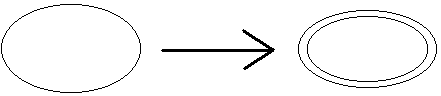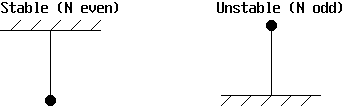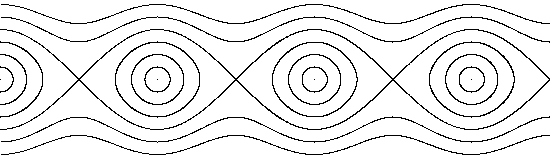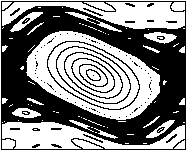# Hamiltonian Chaos

## 10/24/00 Lecture #8 in Physics 505

Warning:  This is probably the most technically difficult lecture of the course.

###Comments on Homework #6 (Lyapunov Exponent)

• Not everyone had a good graph of LE versus C for B = 0.3
• Some had numerical troubles with unbounded orbits (C > 1.42)
• BASIC code for doing part 3 has been put on the WWW

###Review (last week) - Bifurcations

• Bifurcation is a qualitative change in behavior at a critical parameter value
• Observation of a bifurcation verifies determinism
• Flows are often analyzed using their maps (Poincaré section)
• Classifications:
• Local - involves single equilibrium points
• Global - equilibrium points appear or vanish
• Continuous (subtle) - eigenvalues cross unit circle
• Discontinuous (catastrophic) - eigenvalues appear or vanish
• Explosive - like catastrophic but no hysteresis

• (occur when attractor touches the basin boundary)
• There are dozens of bifurcations, many not discovered
• Terminology is not precise or universal (still evolving)
• Transcritical Bifurcation
• Pitchfork Bifurcation
• Flip Bifurcation
• Tangent (or Saddle-Node or Blue Sky) Bifurcation
• Catastrophe (1-D example)
• Hopf Bifurcation
• Niemark (or Secondary Hopf) Bifurcation
• A stable limit cycle becomes unstable and a 2-torus is born

•• The Poincaré section exhibits a Hopf bifurcation
• Main sequence (quasi-periodic route to chaos)
• fixed point --> limit cycle --> 2-torus --> chaos
• N-torus with N > 2 not usually seen (Piexito's Theorem)

• (3-torus and higher are structurally unstable)
• This contradicts the Landau theory of turbulence

• (turbulence is a sum of very many periodic modes)
• Also called the Newhouse-Ruelle-Takens route
• Probably the most common route to chaos at high-D

###Hamiltonian Systems - Introduction and Motivation

• These are systems that conserve mechanical energy
• They have no dissipation (frictionless)
• They are of historical interest and importance
• Examples (all from physics):
• Planetary motion (recall 3-body problem)
• Charged particles in magnetic fields
• Incompressible fluid flows (liquids)
• Trajectories of magnetic field lines
• Quantum mechanics
• Statistical mechanics

###A Case Study - Mass on a Spring (frictionless)

• dx/dt = v
• dv/dt = -(k/m)x
• This system has 1 spatial dimension (1 degree of freedom)
• It has a 2-D phase space however
• Solutionkx2 + mv2 = constant  (conservation of energy)
• HamiltonianH = kx2/2 + mv2/2 (total energy)
• kx2/2 is the potential energy (stored in spring)
• mv2/2 is the kinetic energy (energy of motion)
• Let k = m = 1 for simplicity
• Given the Hamiltonian, we can get the equations of motion:
• dx/dt = dH/dv = v
• dv/dt = -dH/dx = -x
• where d is the partial derivative
• The motion occurs along a 1-D curve in 2-D space
• This curve is not a limit cycle (it is a center)
• Such a system cannot exhibit chaos (even if driven)

###Hamilton's Equations (N Dimensions)

• Generalize the above ideas to dimensions N > 1:
• dqi/dt = dH/dpi  (q is a generalized coordinate)
• dpi/dt = -dH/dqi  (p is a generalized momentum = mv)
• p and q constitute the phase space for the dynamics
• N-dimensional dynamics have a 2N-dimensional phase space
• pi and qi (for i = 1 to N) are the phase space variables
• Note:  dH/dt = dH/dp dp/dt + dH/dq dq/dt = 0
• H is a constant of the motion (Hamiltonian)
• There may be other constants (say a total of k)
• The dynamics are constrained to a 2N - k dimensional space
• Hamiltonian's equations are just another dynamical ODE system:
• dq/dt = f(p, q)
• dp/dt = g(p, q)
• ...
• Note:  dV/dt / V = trace J = fq + gp + ...

• = d/dq [dH/dp] + d/dp [-dH/dq] + ... = 0
• Phase-space volume is conserved

###Properties of Hamiltonian Systems

• They have no dissipation (frictionless)
• There are one or more conserved quantities (energy, ...)
• They are described by a Hamiltonian function H
• There are 2N dimensions for N degrees of freedom
• Motion is on a 2N - k dimensional (hyper)surface
• k + 1 Lyapunov exponents are equal to zero
• There are no attractors (or attractor = basin)
• Transients don't die away (no need to wait)
• Equations are time-reversible
• Orbit returns arbitrarily close to the initial condition
• Phase-space volume is conserved (Liouville's theorem)
• The flow is incompressible (like water)
• The Lyapunov exponents sum to zero
• Chaos can occur only for N > 1 (at least 2 degrees of freedom)
• The dynamics occur in a space of integer dimension
• This space may be a (fat) fractal however (many holes)

###2-D Symplectic (Area-Preserving) Maps

• Xn+1 = f(Xn, Yn)
• Yn+1 = g(Xn, Yn)
• An+1/An = |det J| = |fxgy - fygx| = 1
• Example:  Hénon map with B = 1
• Xn+1 = 1 - CXn2 + Yn
• Yn+1 = Xn
• An+1/An = |0 - (1)(1)| = 1
• Computer demo (C = 0.3)
• More general polynomial symplectic map:
• Xn+1 = A + Yn + F(Xn)
• Yn+1 = B - Xn
• One choice of F is C1 + C2X + C3X2 + ...
• Verify that this has An+1/An = |det J| = 1
• Slide show from Strange Attractors book
• Stochastic web maps:
• These occur for charged particle in EM wave
• Xn+1 = a1 + [Xn + a2sin(a3Yn + a4)]cos a + Ynsin a
• Yn+1 = a5 + [Xn + a2sin(a3Yn + a4)]sin a + Yncos a
• where a = 2p/N  (N is an integer)
• Verify that this has An+1/An = |det J| = 1
• l1 is positive but small
• Exhibit minimal chaos or Arnol'd diffusion
• Examplescase 1 (N = 9) / case 2 (N = 5)

###Simple Pendulum (2-D Conservative Flow)

• dx/dt = v  (v is really an angular velocity)
• dv/dt = -sin x  (x is really an angle)
• For x << 1, sin x --> x and orbits are circles around a center:

•• More generally equilibria are at v* = 0, x* = Np

• (where N is an integer, N = 0, ±1, ±2, ±3, ...)• Phase space trajectories:

•• O-points (centers) and X-points (saddle points)
• Separatrix (homoclinic orbit) separates trapped (elliptic) and passing (hyperbolic) orbits
• Homoclinic orbits are sensitive to perturbations

###Chirikov (Standard) Map

• dx/dt = v
• dv/dt = -sin x
• Solve by the leap-frog method:
• vn+1 = vn - h1sin xn
• xn+1 = xn + h2vn+1
• Leap frog is symplectic if fx = gv = 0
• Let q = x/2p, r = v/2ph1 = Kh2 = 1:
• 2prn+1 = 2prn - K sin(2pqn)
• 2pqn+1 = 2pqn + 2prn+1
• rn+1 = [rn - (K/2p) sin(2pqn)] mod 1
• qn+1 = [qn + rn+1] mod 1
• K is the nonlinearity parameter
• This system also models ball bouncing on vibrating floor
• Animation of Chirikov map

•J. C. Sprott | Physics 505 Home Page | Previous Lecture | Next Lecture